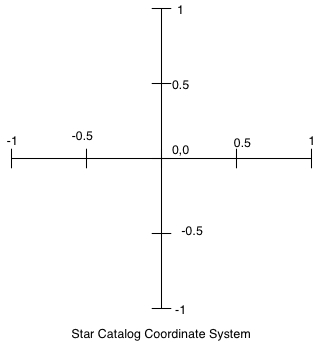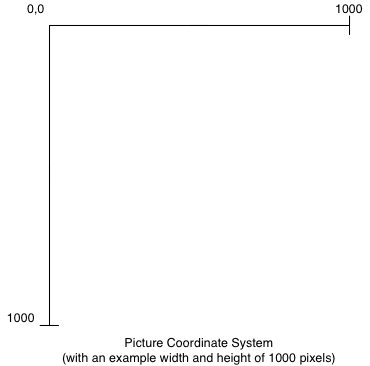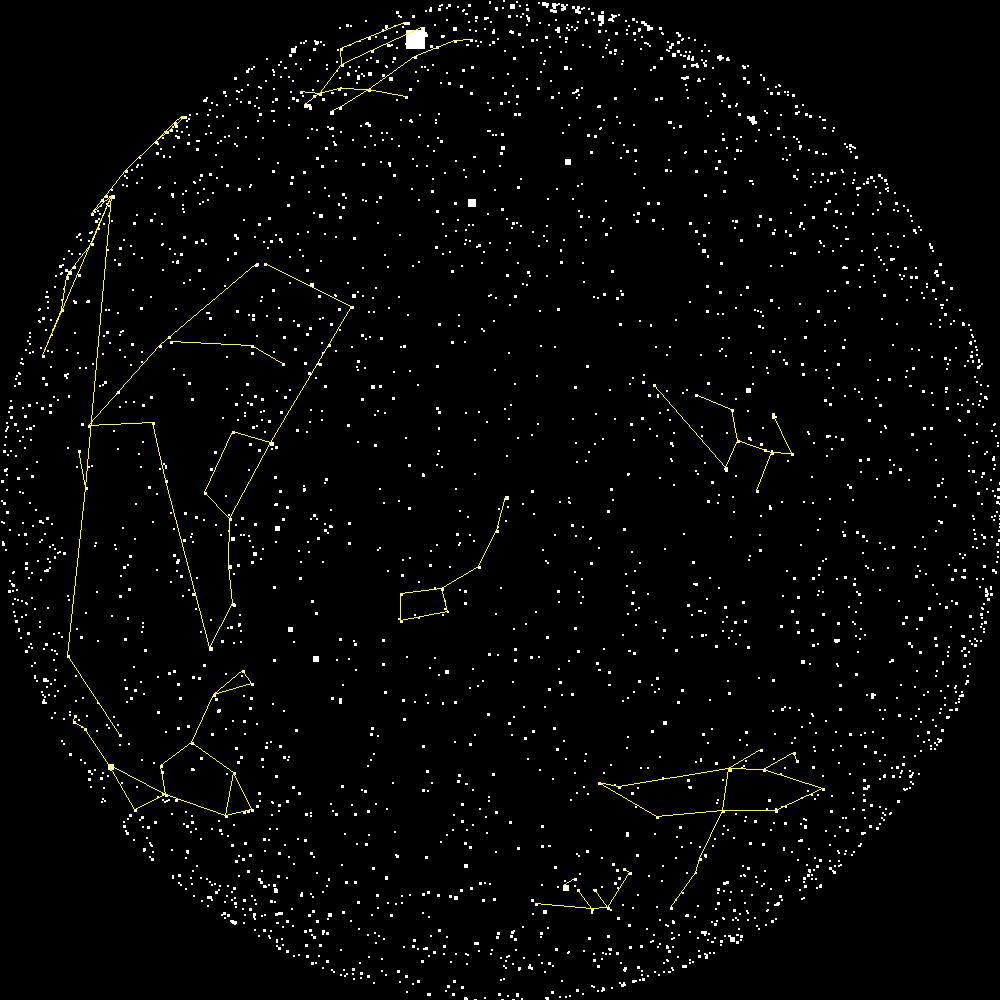Star Charts and Constellations

We had the sky up there, and we used to lay on our backs and look up at them, and discuss whether they was made or just happened.
— Mark Twain

The contemplation of celestial things will make a man both speak and think more sublimely and magnificently when he descends to human affairs.
— Cicero

Overview

The purpose of this assignment is to give you practice in a number of areas:

• Creating, modifying, and extracting data from dictionaries.
• Working with both image and text files.
• Modular development and testing.

Star Catalogs and Coordinate Systems

Astronomers collect lots of data about stars and there are many catalogs that identify the locations of stars. In this assignment, you will use data from a star catalog to create a picture that plots the locations of stars.

Since a real data set often has some incorrect data and the occasional field missing, a cleaned up catalog has been prepared for your use in this assignment. The file stars.txt contains one line for each star that is represented in the catalog. The meaning of each field (column) is described below.

• The first three fields are the x, y and z coordinates for the star. We will ignore the z coordinate, and use only the x and y coordinates. Each axis in the coordinate system goes from -1 to +1, and the centre point is 0,0. (See the figure below.)
• The fourth field is the Henry Draper number, which is simply a unique identifier for the star.
• The fifth field is the magnitude (or brightness) of the star.
• The sixth field is Harvard Revised number, another identifier.
• The seventh field exists only for a small number of stars and is a semicolon-separated list of names for a star. A star may have several names.

Two unique identifiers appear in the data because the star data has been collected from different sources, and the catalogs have several different ways to uniquely identify stars. The fields that you will need for this assignment include the x and y coordinates, the magnitude, the Henry Draper number, and the name (or names) of each star.

The coordinate system used for pixels in a picture has position (0,0) in the upper left corner of the picture, and the maximum x and y values are the height and width of the picture in pixels. In this assignment, all pictures will be square. See below for a comparison of the two coordinate systems.Warmup Functions

To create a picture of the stars, the first thing we need to do is translate from the coordinate system used in the star catalog to the picture coordinate system. The coordinate systems are described above.

Step 1: Translate Coordinates

The first task is to write a function coords_to_pixel(orig_x, orig_y, size): Given the x and y coordinates of a star (original_x and original_y), and the size in pixels of the picture, return the x, y location of the star in terms of pixels in the picture.

For example, if the size of the picture is 1000 by 1000, then the (0, 0) point in the star catalog is at pixel (500, 500), and (0.5, 0.5) from the catalog is at pixel (750, 250).

You should use coords_to_pixel as a helper function in the remainder of the assignment.

Step 2: Draw square ons a picture

Write a function called plot_squares(list) that takes a list of tuples, where each tuple is a point in the coordinate system from the catalog, creates an empty 100 by 100 pixel picture, plots the points as 2 by 2 pixel squares, and returns the picture.

Part 1: Plotting star charts

Implement the following functions in plot_stars.py:

Function Name Description

Given an open text file that contains a star catalog as specified above, return three dictionaries. The first is keyed on the Henry Draper number and the values are tuples containing the x and y coordinates of each star.

The second dictionary is also keyed on the Henry Draper numbers and contains the magnitudes (float) of the stars.

The third dictionary is keyed on the names of the stars and the values are the Henry Draper numbers. If a star has more than one name, each name will appear as a key in the third dictionary. If a star does not have a name it will not be represented in the third dictionary.

plot_plain_stars(picture, int, dict) Given a square picture object, the size of the the picture in pixels, and a dictionary containing the x,y coordinates of stars as values in the dictionary, plot the stars of the picture, and return the picture. Assume that the initial picture is black. The stars are plotted as 2x2 pixel white-filled rectangles. (The add_rect_filled function in the picture module will help you out.)
plot_by_magnitude(picture, int, dict, dict) Given a square picture object, the size of the the picture in pixels, a dictionary containing the x,y coordinates of stars as values in the dictionary, and a dictionary containing the magnitudes, plot the stars on the picture, and return the picture. Assume that the initial picture is black. In this case the stars are plotted as white-filled rectangles, but the size of the rectangle is a function of the magnitude of the stars. Since brighter stars have smaller magnitude values, you will calculate the size of the rectangle as follows:
star_size = round(10.0 / (magnitudes[id] + 2))

Remember to test your code thoroughly. As in previous assignments, you are asked to submit a file test_star_plot.py that contains your test module. Every function you write should have at least one test in the test module, and each test should be specific (only testing one or a small number of conditions), unique (not redundant), and well-documented. You will find it useful to create short catalog files (with only 2 or 3 stars) for testing.

Part 2: Drawing constellations

The image below is an example of the output of your work in this part of the assignment.We are providing several text files that describe that describe constellations. Each file contains two star names per line of the file. Each pair of star names represent one line to be drawn for the constellation. You will write a function to read this data into a dictionary, and add the lines between the stars on to a picture containing a star map.

Constellation files

Download the files of constellation data and implement the following functions in plot_stars.py:

Function Name Description
read_constellation_lines(file) Given an open text file that contains constellation data in the form described above, return a dictionary with star names of type str as keys and lists of star names as values. For each key, the associated list contains all stars connected by a line to the key star.
plot_constellations(Picture, dict, dict, dict, Color, int) Given the following arguments in order:
• a star chart picture,
• a dictionary of coordinates where the keys are Henry Draper numbers, IDs of type str and the values are a tuple of the x, y coordinates.
• a dictionary containing the lines between stars,
• a dictionary containing the mapping between star name and the Henry Draper numbers
• a Color representing the color to draw the lines (Recall that the picture module has many colors defined.)
• an int representing the size of the picture

Plot the lines for the constellation on the picture, and return the picture.

As in Part 1, you may use as many helper functions as you wish. Style and design count, and we expect each function to have at least one test in the test module.